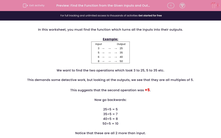# Find the Function from the Given Inputs and Outputs

In this worksheet, students find functions from the given inputs and outputs.Key stage:  KS 2

Curriculum topic:   Maths and Numerical Reasoning

Curriculum subtopic:   Factors and Multiples

Difficulty level:#### Worksheet Overview

In this worksheet, you must find the function which turns all the inputs into their outputs.

Example:

Input   Output
3 25
5 35
6 40
8 50

We want to find the two operations which took 3 to 25, 5 to 35 etc.

This demands some detective work, but looking at the outputs, we see that they are all multiples of 5.

This suggests that the second operation was ×5.

Now go backwards:

25÷5 = 5
35÷5 = 7
40÷5 = 8
50÷5 = 10

Notice that these are all 2 more than input.

The function is therefore  x → (x+2) × 5